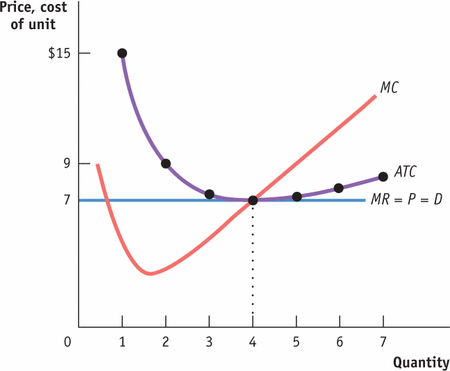Mod 58 Practice Quiz
In order to take this quiz you must be on Duffka.com>C219>Google Forms Quizzes>Mod 58 Practice Quiz
First Name *
Last Name *
Class Period *
1. At what level of output does the firm maximize profit? *
1 point2. At the profit-maximizing quantity of output, is the firm profitable, does it just break even, or does it earn a loss? *
1 point3. At what output does diminishing marginal returns begin?
1 point4. Which curve represents the firms short run supply curve? *
1 point
5. A perfectly competitive firm will maximize profit at the quantity at which the firm’s marginal revenue equals *
1 point
6. Which of the following is correct for a perfectly competitive firm?   I. The marginal revenue curve is the demand curve.   II. The firm maximizes profit when price equals marginal cost.   III. The market demand curve is horizontal. *
1 point
7. A firm is profitable if *
1 point
8. If a firm has a total cost of \$200, its profit-maximizing level of output is 10 units, and it is breaking even (that is, earning a normal profit), what is the market price? *
1 point
9. What is the firm’s profit if the price of its product is \$5 and it produces 500 units of output at a total cost of \$1,000? *
1 point